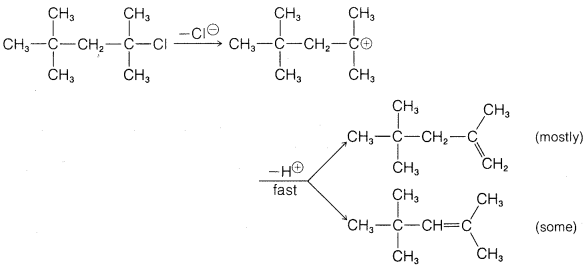# 8.E: Nucleophilic Substitution and Elimination Reactions (Exercises)

Exercise 8-1 Write Lewis structures for each of the following reagents and classify them as either electrophilic, nucleophilic, both, or neither by evaluating whether they will react appreciably with hydroxide ion, $$\ce{HO}^\ominus$$, or hydronium ion, $$\ce{H_3O}^\oplus$$. Write equations for each of the reactions involved.

a. $$\ce{NH_3}$$
b. $$\ce{NH_2^-}$$
c. $$\ce{Na}^\oplus$$
d. $$\ce{Cl}^\ominus$$
e. $$\ce{Cl_2}$$
f. $$\ce{CH_4}$$
g. $$\ce{CN}^\ominus$$
h. $$\ce{CH_3OH}$$
i. $$\ce{CH_3} \overset{\oplus}{\ce{O}} \ce{H_2}$$
j. $$\ce{BF_4^-}$$
k. $$\ce{HBr}$$
l. $$\ce{HC \equiv C} \colon^\ominus$$
m. $$\colon \ce{CH_2}$$
n. $$\ce{FSO_3H}$$
o. $$\ce{SO_3}$$

Exercise 8-2 Identify the electrophile and the nucleophile in each of the following reactions:

a. $$\ce{CH_3I} + \overset{\ominus}{\ce{O}} \ce{CH_3} \rightarrow \ce{CH_3-O-CH_3} + \ce{I}^\ominus$$

b. $$\ce{CH_2=CH_2} + \ce{Br_2} \rightarrow \overset{\oplus}{\ce{C}} \ce{H_2-CH_2-Br} + \ce{Br}^\ominus$$

c. $$\ce{CH_3NH_2} + \ce{CH_3I} \rightarrow \ce{(CH_3)_2NH} + \ce{HI}$$

Exercise 8-3 Calculate $$\Delta H^0$$ for the polar reaction of one mole of bromomethane with water in accord with the equation $$\ce{CH_3Br} + \ce{H_2O} \rightarrow \ce{CH_3OH} + \ce{HBr}$$ (a) in the gas phase and (b) with all of the participants in dilute aqueous solution. For Part (a) you will need the bond energies of Table 4-3 and for Part (b) you will require the bond energies and the following $$\Delta H^0$$ values:

Exercise 8-4 Ethyl chloride $$\left( 0.1 \: \text{M} \right)$$ reacts with potassium iodide $$\left( 0.1 \: \text{M} \right)$$ in 2-propanone (acetone) solution at $$60^\text{o}$$ to form ethyl iodide and potassium chloride at a rate $$\left( v \right)$$ of $$5.44 \times 10^{-7} \: \text{mol L}^{-1} \: \text{s}^{-1}$$.

a. If the reaction proceeded by an $$S_\text{N}2$$ mechanism, what is the value of $$k$$ (in proper units) and what would be the rate of the reaction in moles per liter per sec at $$0.01 \: \text{M}$$ concentrations of both reactants? Show your method of calculation.

b. Suppose the rate were proportional to the square of the potassium iodide concentration and the first power of the ethyl chloride concentration. What would be the rate with $$0.01 \: \text{M}$$ reactants?

c. It one starts with solutions initially $$0.1 \: \text{M}$$ in both reactants, the rate of formation of ethyl iodide initially is $$5.44 \times 10^{-7} \: \text{mol L}^{-1} \: \text{s}^{-1}$$, but falls as the reaction proceeds and the reactants are used up. Plot the rate of formation of ethyl iodide against the concentration of ethyl chloride as the reaction proceeds (remembering that one molecule of ethyl chloride consumes one molecule of potassium iodide). Assume that the rate of reaction is proportional to the first power of the ethyl chloride concentration; and to (1) the zeroth power, (2) the first power, and (3) the second power of the potassium iodide concentration.

d. What kind of experimental data would one need to determine whether the rate of the reaction of ethyl chloride with potassium iodide is first order in each reactant or second order in ethyl chloride and zero order in potassium iodide?

Exercise 8-5 The rate of solvolysis of tert-butyl chloride in aqueous solution is unaffected by having sodium azide, $$\overset{\oplus}{\ce{Na}} \overset{\ominus}{\ce{N}} \ce{=} \overset{\oplus}{\ce{N}} \ce{=} \overset{\oplus}{\ce{N}}$$, in the solution, yet the products include both 2-azido-2-methylpropane, $$1$$, and tert-butyl alcohol:Show how this information can be used to determine whether an $$S_\text{N}1$$ or an $$S_\text{N}2$$ mechanism occurs in the solvolysis of tert-butyl chloride in aqueous solution.

Exercise 8-6 What inference as to reaction mechanism might you make from the observation that the rate of hydrolysis of a certain alkyl chloride in aqueous 2-propanone is retarded by having a moderate concentration of lithium chloride in the solution?

Exercise 8-7 Equations 8-3 through 8-5 show how Kenyon and Phillips established that inversion of configuration accompanies what we now recognize to be $$S_\text{N}2$$ substitutions. For each reaction, we indicate whether $$\ce{R-O}$$ or $$\ce{O-H}$$ is broken by an appropriately placed vertical line. Explain how the sequence of steps shows that inversion occurs in the $$S_\text{N}2$$ reaction of Equation 8-5. The symbols $$\left( + \right)$$ or $$\left( - \right)$$ designate for each compounds the sign of the rotation $$\alpha$$ of the plane of polarized light that it produces.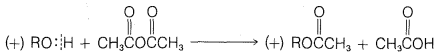$$\tag{8-3}$$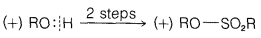$$\tag{8-4}$$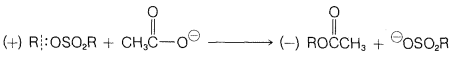$$\tag{8-5}$$

Exercise 8-8 Explain how, in the presence of bromide ion, either enantiomer of 2-bromobutane racemizes (Section 5-1B) in 2-propanone solution at a rate that is first order in $$\ce{Br}^\ominus$$ and first order in 2-bromobutane.

Exercise 8-9* When either of the enantiomers of 1-deuterio-1-bromobutane is heated with bromide ion in 2-propanone, it undergoes an $$S_\text{N}2$$ reaction that results in a slow loss of its optical activity. If radioactive bromide ion $$\left( \ce{Br}^* \: ^\ominus \right)$$ is present in the solution, radioactive 1-deuterio-1-bromobutane is formed by the same $$S_\text{N}2$$ mechanism in accord with the following equation:

$\ce{CH_3CH_2CH_2CHDBr} + \ce{Br}^* \: ^\ominus \overset{\text{2-propanone}}{\longrightarrow} \ce{CH_3CH_2CH_2CHDBr}^* + \ce{Br}^\ominus$

Within experimental error, the time required to lose $$10\%$$ of the optical activity is just equal to the time required to have $$5\%$$ of the $$\ce{CH_3CH_2CH_2CHDBr}$$ molecules converted to $$\ce{CH_3CH_2CH_2CHDBr}^*$$ with radioactive bromide ion. Explain what we can conclude from these results as to the degree to which the $$S_\text{N}2$$ reaction produces inversion of configuration of the primary carbon of 1-deuterio-1-bromobutane.

Exercise 8-10 What can be concluded about the mechanism of the solvolysis of 1-butyl derivatives in ethanoic acid from the projection formulas of the starting material and product of the following reaction?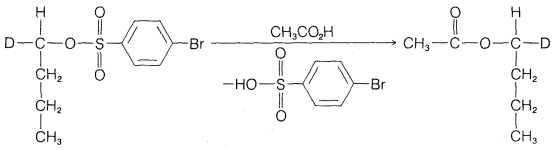Would you call this an $$S_\text{N}1$$ or $$S_\text{N}2$$ reaction?

Exercise 8-11 In the reaction of 1-phenylethanol with concentrated $$\ce{HCl}$$, 1-phenylethyl chloride is formed:If the alcohol originally has the $$D$$ configuration, what configuration would the resulting chloride have if formed (a) by the $$S_\text{N}2$$ mechanism and (b) by the $$S_\text{N}1$$ mechanism?

Exercise 8-12 Predict which compound in each of the following groups reacts most rapidly with potassium iodide in 2-propanone as solvent by the $$S_\text{N}2$$ mechanism. Give your reasoning and name the substitution product by the IUPAC system.

a. $$\ce{(CH_3)_3CCH_2Cl}$$ $$\ce{(CH_3)_3CCl}$$ $$\ce{CH_3CH_2CH_2CH_2Cl}$$

b.c.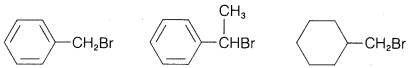Exercise 8-13 Which of the monobromine-substituted methylcyclohexanes would you judge to be the most reactive in (a) $$S_\text{N}2$$-type displacement and (b) $$S_\text{N}1$$-type displacement?

Exercise 8-14 Answer the question in the preceding exercise, but with the monobromine-substituted 1-methylcyclohexenes.

Exercise 8-15 Select the compounds from the following list that would be expected to hydrolyze more rapidly than phenylmethyl (benzyl) chloride by the $$S_\text{N}1$$ mechanism:

a. 2-phenylmethyl chloride
b. diphenylmethyl chloride
c. 1-phenylmethyl chloride
d. (chloromethyl)cyclohexane
e. 1-chloro-4-methylbenzene

Exercise 8-16 Explain the following observations:

a. The tertiary chloride, apocamphyl chloride, is unreactive in either $$S_\text{N}1$$ or $$S_\text{N}2$$ reactions. For example, no reaction occurs when its solution in aqueous ethanol containing $$30\%$$ potassium hydroxide is refluxed for 20 hours.b. Chloromethyl alkyl (or aryl) ethers, $$\ce{ROCH_2Cl}$$, are very reactive in $$S_\text{N}1$$ solvolysis reactions. Compared to chloromethane, the rate of hydrolysis of chloromethyl phenyl ether is about $$10^{14}$$. Also, the rate of hydrolysis is retarded significantly by lithium chloride.

Exercise 8-17 Methyl sulfides are prepared conveniently by the $$S_\text{N}2$$ reaction of a $$\ce{CH_3X}$$ derivative with a sulfur nucleophile: $$\ce{RS}^\ominus + \ce{CH_3X} \rightarrow \ce{RSCH_3} + \ce{X}^\ominus$$. The rate of the reaction with a given $$\ce{RS}^\ominus$$. If you are uncertain of the p$$K_a$$ of the acids $$\ce{HX}$$, look them up in an appropriate reference, such as the CRC Handbook of Physics and Chemistry.

a. dimethyl sulfate, $$\ce{(CH_3O)_2SO_2}$$
b. methyl nitrate, $$\ce{CH_3ONO_2}$$
c. methyl cyanide (ethanenitrile), $$\ce{CH_3CN}$$
d. methyl fluoride, $$\ce{CH_3F}$$
e. methyl iodide, $$\ce{CH_3I}$$
f. methyl fluorosulfonate,g. methyl acetate (ethanoate),h. methanol, $$\ce{CH_3OH}$$

Exercise 8-18 Account for the following observations:

a. tert-Alkyl fluorides are unreactive in $$S_\text{N}1$$ solvolysis reactions unless a strong acid is present.

b. $$D$$-1-Phenylethyl chloride dissolved in aqueous 2-propanone containing mercuric chloride loses much of its optical activity before undergoing hydrolysis to give racemic 1-phenylethanol.

c. 1-Bromobutane can be prepared by heating 1-butanol with a mixture of sodium bromide and sulfuric acid. The reaction fails, however, if the sulfuric acid is omitted.

d. Benzenoxide (phenoxide) ion, $$\ce{C_6H_5O}^\ominus$$, is a better leaving group than ethoxide, $$\ce{C_2H_5O}^\ominus$$.

Exercise 8-19 Using the discussion in Section 8-7 of how the structure of $$\ce{R}$$ and $$\ce{X}$$ influence the $$S_\text{N}$$ reactivity of $$\ce{RX}$$, predict the favored course of each of the following reactions. Give your reasoning.

a.b. $$\ce{(CH_3)_3C-O-CH_3} + \ce{HI} \rightarrow$$

c. $$\ce{CF_3-O-CH_3} + \ce{HBr} \rightarrow$$

d.Exercise 8-20 Methyl ethers of the type $$\ce{R-O-CH_3}$$ cannot be prepared by the reaction of the alcohol $$\ce{ROH}$$ with $$\ce{CH_3I}$$, but if $$\ce{Ag_2O}$$ is present the following reaction occurs under mild conditions:

$2 \ce{R-OH} + \ce{Ag_2O} + 2 \ce{CH_3I} \rightarrow 2 \ce{ROCH_3} + 2 \ce{AgI} + \ce{H_2O}$

Explain how $$\ce{Ag_2O}$$ promotes this reaction.

Exercise 8-21 Explain each of the following observations:

a. Methyl sulfide $$\ce{(CH_3)_2S}$$ reacts with $$\ce{C_6H_5COCH_2Cl}$$ in benzene to give the sulfonium salt, $$\ce{C_6H_5COCH_2} \overset{\oplus}{\ce{S}} \ce{(CH_3)_2Cl}^\ominus$$, which precipitates as it is formed. Attempts to recrystallize the product from ethanol result in formation of methyl sulfide and $$\ce{C_6H_5COCH_2Cl}$$.

b. $$S_\text{N}2$$ displacements of alkyl chlorides by $$\overset{\ominus}{\ce{O}} \ce{H}$$ often are catalyzed by iodide ion, $$\ce{RCl} + \colon \overset{\ominus}{\ce{O}} \ce{H} \overset{\ce{I}^\ominus}{\longrightarrow} \ce{ROH} + \ce{Cl}^\ominus$$ and may result in a product with less than $$100\%$$ of inverted configuration at the carbon carrying the chlorine.

c.* Tris(trifluoromethyl)amine, $$\ce{(CF_3)_3N}$$, is completely nonnucleophilic, whereas trimethylamine is a good nucleophile.

Exercise 8-22 Classify the following solvents according to effectiveness for solvation of (i) cations and (ii) anions:

a. 2-propanone, $$\ce{CH_3COCH_3}$$
b. tetrachloromethane, $$\ce{CCl_4}$$
c. anhydrous hydrogen fluoride, $$\ce{HF}$$
d. trichloromethane, $$\ce{CHCl_3}$$
e. trimethylamine, $$\ce{(CH_3)_3N}$$
f. trimethylamine oxide, $$\ce{(CH_3)_3} \overset{\oplus}{\ce{N}} - \overset{\ominus}{\ce{O}}$$

Exercise 8-23* Would you expect the $$S_\text{N}2$$ reaction of sodium cyanide with methyl bromide to be faster, slower, or about the same with $$\ce{(CH_3)_2S=O}$$ or ethanol as solvent? Explain.

Exercise 8-24 An alternative mechanism for $$E2$$ elimination is the following:

$\ce{CH_3CH_2Cl} + \ce{OH}^\ominus \overset{\text{fast}}{\rightleftharpoons} \: ^\ominus \colon \ce{CH_2CH_2Cl} + \ce{H_2O} \overset{\text{slow}}{\longrightarrow} \ce{CH_2=CH_2} + \ce{Cl}^\ominus$

a. Would this mechanism lead to overall second-order kinetics with respect to the concentrations of $$\ce{OH}^\ominus$$ and ethyl chloride? Explain.

b. This mechanism as written has been excluded for several halides by carrying out the reaction in deuterated solvents such as $$\ce{D_2O}$$ and $$\ce{C_2H_5OD}$$. Explain how such experiments could be relevant to the reaction mechanism.

c. Does the test in Part b also rule out $$\ce{CH_3CH_2Cl} + \overset{\ominus}{\ce{O}} \ce{H} \overset{\text{slow}}{\longrightarrow} \colon \overset{\ominus}{\ce{C}} \ce{H_2CH_2Cl} + \ce{H_2O} \overset{\text{fast}}{\longrightarrow} \ce{CH_2=CH_2} + \ce{Cl}^\ominus$$? Explain.

Exercise 8-25 Write equations and mechanisms for all the products that might reasonably be expected from the reaction of 2-chlorobutane with a solution of potassium hydroxide in ethanol.

Exercise 8-26

a. Why is potassium tert-butoxide, $$\overset{\oplus}{\ce{K}} \overset{\ominus}{\ce{O}} \ce{C(CH_3)_3}$$, an excellent base for promoting elimination reactions of alkyl halides, whereas ethylamine, $$\ce{CH_3CH_2NH_2}$$, is relatively poor for the same purpose?

b. Potassium tert-butoxide is many powers of ten more effective a reagent for achieving $$E2$$ eliminations in methylsulfinylmethane (dimethyl sulfoxide) than in tert-butyl alcohol. Explain.

Exercise 8-27 Which one of the following groups of compounds would eliminate $$\ce{HCl}$$ most readily on reaction with potassium hydroxide? Draw the structure of the product and name it.

a. $$\ce{(CH_3)_3CCl}$$ $$\ce{CH_3CH_2CH_2CH_2Cl}$$ $$\ce{CH_3CH(Cl)CH_2CH_3}$$

b. $$\ce{(CH_3)_3CCH_2Cl}$$ $$\ce{(CH_3)_2CHCH_2Cl}$$
c.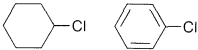Exercise 8-28 Which one of each of the following pairs of compounds would react most rapidly with potassium hydroxide in an $$E2$$-type elimination? Draw the structure of the product and name it.

a. $$\ce{(CH_3)_3COH}$$ $$\ce{(CH_3)_3CCl}$$

b.c.Exercise 8-29 Write all the possible staggered conformations for each of the isomers of 2,3-dibromobutane, $$8$$ and $$9$$: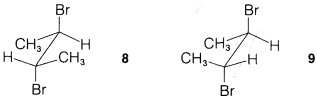Show the structures of the alkenes that could be formed from each by antarafacial $$E2$$ elimination of one mole of hydrogen bromide with hydroxide ion. Which alkene should more readily eliminate further to form 2-butyne? Explain.

Exercise 8-30 For the reaction of Equation 8-7, would you expect the ratio of tert-butyl alcohol to 2-methylpropene to change significantly with changes in the nature of the leaving group [i.e., $$\ce{Cl}$$, $$\ce{Br}$$, $$\ce{I}$$, or $$\overset{\oplus}{\ce{S}} \ce{(CH_3)_2}$$]? Give your reasoning.

Would you expect the same or different behavior as $$\ce{X}$$ is changed, if elimination were occurring by an $$E2$$ mechanism with the solvent acting as the base? Explain.

Exercise 8-31 The reaction of tert-butyl chloride with water is accelerated strongly by sodium hydroxide. How would the ratio of elimination to substitution products be affected thereby? Explain.

Exercise 8-32 Explain how $$\ce{(CH_3)_2CDCHBrCH_3}$$ (where $$\ce{D}$$ is the hydrogen isotope of mass 2) might be used to determine whether 2-methyl-2-butene is formed directly from the bromide in an $$E1$$ reaction, or by rearrangement and elimination as shown in the preceding equations.

Exercise 8-33 Predict the products of the following reactions:

a. $$\ce{CH_3CH_2CBr(CH_3)CH_2CH_3} \underset{S_\text{N}1, \: E1}{\overset{\ce{H_2O}}{\longrightarrow}}$$

b. $$\ce{(CH_3)_3CCH(CH_3)Cl} \underset{S_\text{N}1, \: E1}{\overset{\ce{H_2O}}{\longrightarrow}}$$

c.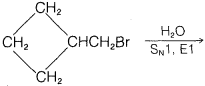Exercise 8-34 Write reaction sequences, using specific and appropriate compounds, that illustrate the following conversions:

a. alcohol $$\rightarrow$$ ether
b. alcohol $$\rightarrow$$ alkene
c. alcohol $$\rightarrow$$ alkyl chloride
d. alcohol $$\rightarrow$$ nitrile $$\left( \ce{ROH} \rightarrow \ce{RCN} \right)$$
e. alkyl chloride $$\rightarrow$$ sulfonium salt $$\rightarrow$$ alkene

Exercise 8-35 $$\ce{S}$$-Adenosylmethionine is a biologically important compound that reacts in the $$S_\text{N}2$$ manner with the amino group of phosphorylated 2-aminoethanol, $$\ce{NH_2CH_2CH_2OPO_3H_2}$$. Which carbon of $$\ce{S}$$-adenosylmethionine would be most likely to undergo an $$S_\text{N}2$$ reaction with an $$\ce{RNH_2}$$ compound? Give your reasoning and write the structures of the expected products.Exercise 8-36 Nitriles, $$\ce{RCN}$$, can be prepared by $$S_\text{N}2$$ displacement of alkyl derivatives, $$\ce{RX}$$, by using sodium or potassium cyanide:

$\ce{RX} + \ce{NaCN} \rightarrow \ce{RCN} + \ce{NaX}$

a. Which of the following solvents would be most suitable for this reaction: water, 2-propanone, ethanol, benzene, $$\ce{(CH_3)_2S=O}$$, or pentane? Give reasons for your choice.

b. Which of the six isomeric monobromoderivatives of 1-methylcyclohexane would you expect to react most rapidly with sodium cyanide? Why?

c. If you wished to make 2-phenylethanenitrile, $$\ce{C_6H_5CH_2CN}$$, which of the following phenylmethyl compounds, $$\ce{RCH_2X}$$, would you select to convert to the nitrile? $$\ce{X} = \ce{-F}$$, $$\ce{-OH}$$, $$\ce{-OCOCH_3}$$, $$\ce{-H}$$, $$\ce{-NH_2}$$, $$\ce{-O_3SCH_3}$$, $$\ce{-SO_3-CH_3}$$. Why?

Exercise 8-37 Give a plausible explanation for each of the following observations:

a. Aqueous sodium chloride will not convert tert-butyl alcohol to tert-butyl chloride but concentrated hydrochloric acid will.

b. Better yields are obtained in the synthesis of isopropyl methyl ether starting with methyl iodide rather than sodium methoxide:

$\ce{CH_3I} + \ce{(CH_3)_2CHO}^\ominus \ce{Na}^\oplus \rightarrow \ce{(CH_3)_2CHOCH_3} + \ce{Na}^\oplus \ce{I}^\ominus$

$\ce{(CH_3)_2CHI} + \ce{CH_3O}^\ominus \ce{Na}^\oplus \rightarrow \ce{(CH_3)_2CHOCH_3} + \ce{Na}^\oplus \ce{I}^\ominus$

c. The following reaction proceeds only if an equivalent amount of silver fluoroborate, $$\ce{Ag}^\oplus \ce{BF_4} \: ^\ominus$$, is added to the reaction mixture:d. 1-Bromo-2-butene reacts with water to give a mixture of 2-buten-1-ol, 3-buten-2-ol, and some 1,3-butadiene.

Exercise 8-38 Which compound in the following pairs would react faster under the reaction conditions? Draw the structures of the major products expected.

a. $$\ce{C_6H_5CH_2CH_2Br}$$ or $$\ce{C_6H_5C(CH_3)(Br)CH_3}$$ in ethanol-water solution.

b. Same as in Part a, but with potassium iodide in acetone.

c. Same as in Part a, but with potassium hydroxide in ethanol.

d. $$\ce{CH_3CH_2} \overset{\oplus}{\ce{N}} \ce{(CH_3)_3} \: \overset{\ominus}{\ce{B}} \ce{F_4}$$ or $$\ce{CH_3CH_2} \overset{\oplus}{\ce{N}} \ce{(CH_3)_3} \: \overset{\ominus}{\ce{O}} \ce{CH_3}$$ on heating in methanol solution.

Exercise 8-39 Show the products of the following reactions and indicate the stereochemistry where important.

a. trans-1-bromo-3-methylcyclopentane $$\underset{\text{acetone}}{\overset{\ce{KI}}{\longrightarrow}}$$

b. trans-1-chloro-2-methylcyclopentane $$\underset{\textit{tert} \text{-butyl alcohol}}{\overset{^\oplus \ce{K} ^\ominus \ce{O} \ce{C(CH_3)_3}}{\longrightarrow}}$$

c.d. $$D$$-2-butanol $$\overset{\text{potassium metal}}{\longrightarrow}$$ potassium salt $$\overset{L \text{-2-chlorobutane}}{\longrightarrow}$$

e.f. meso-1,2-dibromo-1,2-diphenylethane $$\overset{^\oplus \ce{K} ^\ominus \ce{O} \ce{C(CH_3)_3}}{\longrightarrow}$$

Exercise 8-40 The $$S_\text{N}1$$ reactions of many $$\ce{RX}$$ derivatives that form moderately stable carbocations are substantially retarded by adding $$\ce{X}^\ominus$$ ions. However, such retardation is diminished, at given $$\ce{X}^\ominus$$ concentrations, by adding another nucleophile such as $$\ce{N_3} \: ^\ominus$$. Explain.

Exercise 8-41 The reaction of 1-chlorobutane with sodium hydroxide to give 1-butanol is catalyzed by sodium iodide.

a. Work out the stereochemistry to be expected for both the catalyzed and the uncatalyzed reactions if(optically active) were used as the starting material. Show your reasoning.

b. Does retention of configuration, as the overall result of an $$S_\text{N}2$$, automatically preclude operation of the usual mechanism? Explain.

Exercise 8-42* Suppose a water solution was made up initially to be $$0.01 \: \text{M}$$ in methyl bromide and $$1.0 \: \text{M}$$ in sodium ethanoate at $$50^\text{o}$$. In water, the $$S_\text{N}2$$ rate constant for reaction of hydroxide ion with methyl bromide at $$50^\text{o}$$ is $$30 \times 10^{-4} \: \text{L mol}^{-1} \: \text{sec}^{-1}$$, whereas that of ethanoate ion at $$50^\text{o}$$ is $$1.0 \times 10^{-4} \: \text{L mol}^{-1} \: \text{sec}^{-1}$$. The ionization constant of ethanoic acid at $$50^\text{o}$$ is $$1.8 \times 10^{-5}$$. In the following, neglect the rates of the reactions of methyl bromide with water or ethanoic acid and any further reactions of ethanoate:

a. Calculate the hydroxide-ion concentration in the initial solution.

b. Calculate the initial rates of formation of methyl ethanoate and methanol.

c. Compute the concentrations of the organic products when the reaction is complete. Show your reasoning and justify any assumptions.

d. What kind of information would be needed to predict what products would be expected from a solution of methyl bromide and sodium hydroxide in methanol? Explain.

Exercise 8-43 Indicate how you would synthesize each of the following substances from the given organic starting materials and any other necessary organic or inorganic reagents. Specify reagents and conditions. (You may have to use reactions discussed in Chapter 4.)

a. $$\ce{CH_2=CH-CH_2OCOCH_3}$$ from propene
b. $$\ce{CH_3-O-CH_2CH_3}$$ from ethanol
c. $$\ce{CH_3-O-C(CH_3)_3}$$ from tert-butyl alcohol
d. cyclohexene from cyclohexane

Exercise 8-44 Which compound in each of the following pairs would you expect to react more readily with (A) potassium iodide in 2-propanone, (B) concentrated sodium hydroxide in ethanol, and (C) silver nitrate in aqueous ethanol? Write equations for all the reactions involved and give your reasoning with respect to the predicted orders of reactivity.

a. methyl chloride and isobutyl chloride with A, B, and C
b. methyl chloride and tert-butyl chloride with A, B, and C
c. tert-butyl chloride and 1-fluoro-2-chloro-2-methylpropane with B and C
d. 1-chloro-2-butene and 4-chloro-1-butene with A, B, and C

Exercise 8-45 Classify each of the following reactions from the standpoint of yield, side reactions, and reaction rate as good, fair, or bad synthetic procedures for preparation of the indicated products under the given conditions. Show your reasoning and designate any important side reactions.

a.b.c.d.e.f.g.h.Exercise 8-46 Consider each of the following compounds to be in unlabeled bottles in pairs as indicated. For each pair give a chemical test (preferably a test-tube reaction) that will distinguish between the two substances. Write equations for the reactions involved.

$\begin{array}{lll} & \textit{Bottle A} & \textit{Bottle B} \\ \textbf{a.} & \ce{(CH_3)_3CCH_2Cl} & \ce{CH_3CH_2CH_2CH_2Cl} \\ \textbf{b.} & \ce{BrCH=CHCH_2Cl} & \ce{ClCH=CHCH_2Br} \\ \textbf{c.} & \ce{(CH_3)_3CCl} & \ce{(CH_3)_2CHCH_2Cl} \\ \textbf{d.} & \ce{CH_3CH=CHCl} & \ce{CH_2=CHCH_2Cl} \\ \textbf{e.} & \ce{(CH_3)_2C=CHCl} & \ce{CH_3CH_2CH=CHCl} \\ \textbf{f.} & \ce{CH_3CH_2CH=CHCl} & \ce{CH_2=CHCH_2CH_2Cl} \end{array}$

Exercise 8-47 Why does the following $$E1$$ reaction give more of the least substituted alkene? (Use models.)